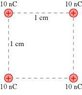# Electric potential of four charges

Metalsonic75
There are four spheres arranged as the four corners of a square, each sphere having a charge of 10 nC and separated from each other by a distance of 1 cm. The four 1.0 g spheres are released simultaneously and allowed to move away from each other. What is the speed of each sphere when they are very far apart? (see attached figure).

I know that for kinetic and potential energy deltaK = -deltaU
K_f - K_i = U_f - U_i
K_i = 0
U_f = 0

K_f = U_i

First I found the total potential energy of the system. U = (q_1*q_2*k)/r. Since all charges are the same, the interaction of any two charges is q^2 (where q^2 = 1*10^-16). The six interactions between the charges produce the following calculation for potential energy:
U = K { q^2/r + q^2/r + q^2/r +q^2/r + q^2/sqrt(2)r + q^2/sqrt(2)r }
U = K { 4q^2/r + 2q^2/sqrt(2)r) }
Plugging in 10^-8 for q, and 0.01 for r, gets
U = K (5.414*10^-14)
U = 0.000487

Since U_i = K_f,
0.000487 = 0.5mv^2
The mass of the spheres is 1g (0.001 kg)
0.973 = v^2
v = 0.987, which should be the final speed of each sphere. This is incorrect, though.

Could somebody please tell me where I went wrong? Thank you for your time.

#### Attachments

•knight_Figure_29_78.jpg
3.9 KB · Views: 1,118

Homework Helper
Hi Metalsonic75,

Since U_i = K_f,
0.000487 = 0.5mv^2
The mass of the spheres is 1g (0.001 kg)
0.973 = v^2
v = 0.987, which should be the final speed of each sphere. This is incorrect, though.

Could somebody please tell me where I went wrong? Thank you for your time.

I can't see your attachment yet, but I think the equation in bold is incorrect; can you see why? Remember that the potential energy term on the left was from all four particles.

Metalsonic75
QUOTE: Remember that the potential energy term on the left was from all four particles.

Ah! Thank you! I now have the correct answer!